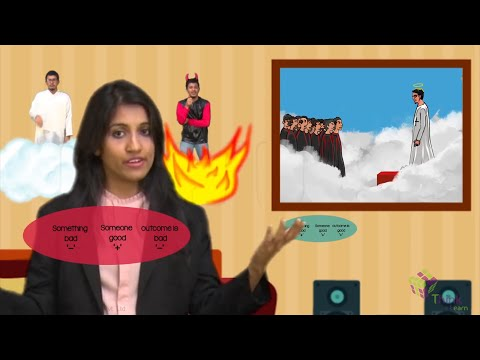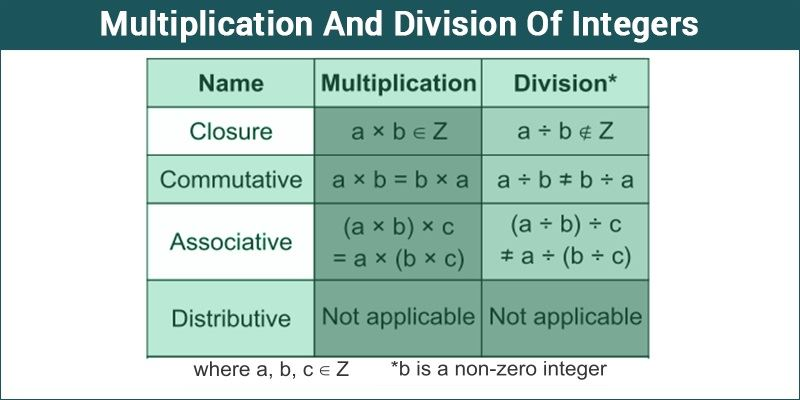Multiplication And Division Of IntegersThe arithmetic operation is a branch of Mathematics that involves addition, subtraction, division and multiplication on all types of real numbers including integers.

Integers are a particular kind of numbers that include negative numbers, positive numbers and zero and no fractions. Arithmetic operations on integers and real numbers is similar that can be either positive or negative.

Rules for multiplication and division of integers are explained below.MULTIPLICATION OF INTEGERS

By definition, multiplication is the repeated addition of numbers. But the rules for multiplication of integers are different from that of addition. It includes three possibilities. They are:

• Multiplication between two positive numbers,
• Multiplication between two negative numbers; and
• Multiplication between a positive number and a negative number.

The product of two integers with similar sign numbers will always be positive. This means, product of two positive numbers or two negative numbers will always be positive. While the product of a positive number and a negative number (integers with different signs) will always be negative.

Rules of Multiplication

 Type of  Numbers Operation Result Example Positive * Positive Multiply Positive (+) 1 * 5 = 5 Negative * Negative Multiply Positive (+) (-1) * (-5) = 5 Positive * Negative Multiply Negative (-) 1 * (-5) = -5 Negative * Positive Multiply Negative (-) (-1) * 5 = -5

In case of multiplication of integers, just multiply the numbers without sign. Once the product is obtained give the sign according to the rule of multiplication given in above table. Since multiplication is commutative property i.e. -4 $\times$ 3 = 3 $\times$ -4 the result will be same .Hence, the 3rd and 4th rules in the table are equivalent.
To avoid the confusion, let’s picture the scenario as below:

• When something good (+) happens to someone good (+), it is Good (+).
• When something good (+) happens to someone bad (-), it is Bad (-).
• When something bad (-) happens to someone good (+), it is Bad (-).
• When something bad (-) happens to someone bad (-), it is Good (+).

Note:

• If number of negative integers to multiply is odd, the product will be negative

(Ex:$-2 \times -4 \times -3~ =~-24$).

• If number of negative integers to multiply is even, the product will be positive

(Ex:$-4 \times -3~=~-12$))

DIVISION OF INTEGERS

If multiplication is totaling of numbers, division is the distribution of numbers. Dividing integers is opposite operation of multiplication. But the rules for division of integers are same as multiplication rules.Though, it is not always necessary that the quotient will always be an integer.

Rule 1: The quotient of two positive integers will always be positive.

Rule 2: The quotient of two negative integers will always be positive.

Rule 3: The quotient of a positive integer and a negative integer will always be negative.

Rules for Dividing Integers

 Type of  Numbers Operation Result Example Positive ÷ Positive Divide Positive (+) 10 ÷ 5 = 2 Negative ÷ Negative Divide Positive (+) (-10) ÷ (-5) = 2 Positive ÷ Negative Divide Negative (-) 10 ÷ (-5) = -2 Negative ÷ Positive Divide Negative (-) (-10) ÷ 5 = -2

Same as multiplication, divide the integers without sign, then give the sign according to the rule as given in the table. The division of two integers with the like signs gives positive quotient and the division of two integers with unlike signs gives negative quotient.

To solve more problems on the topic, download Byju’s – The Learning App from Google Play Store and watch interactive videos. Also, take free tests to practice for exams.